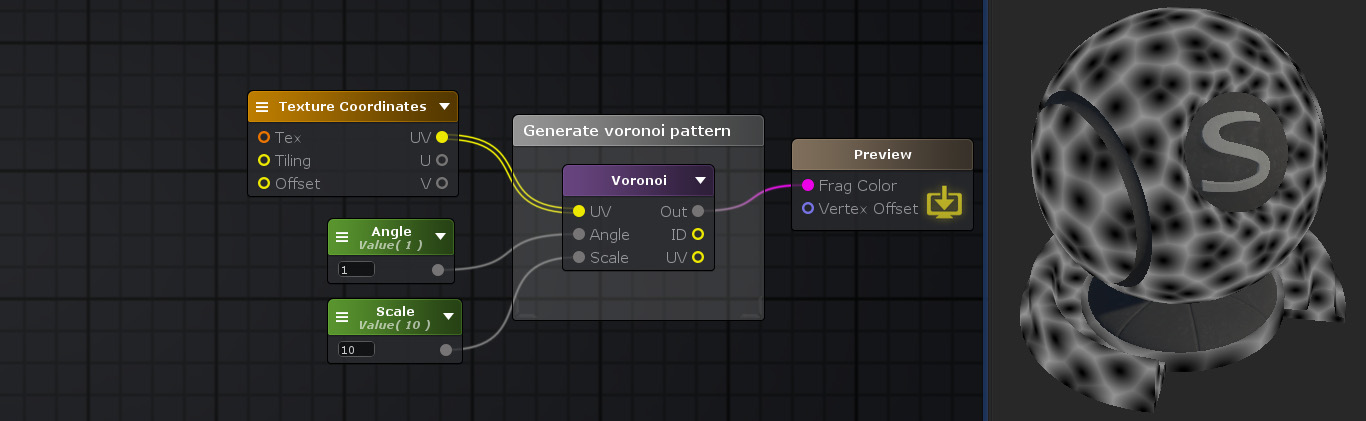(diff) ← Older revision | Latest revision (diff) | Newer revision → (diff)

## Voronoi Node

The Voronoi node creates a voronoi pattern value under a [0 1] range based using the method specified by Type according to the values specified by UV, Angle and Scale.
NOTE: Input data must vary across the the geometry since equal values will generate the same voronoi value. A simply way to achieve this is to connect a Texture Coordinates node into its input.Nodes used: Texture Coordinate, Float, Voronoi

Node Parameter Description Default Value
Method Method used to generate the voronoi pattern
• Cells:
• Cristal:
• Glass:
• Caustics:
• Distance:
Cells
Distance Function Pattern is calculated via the distance to each seed. Each distance function generates different results on top of the same voronoi type.
• Euclidian^2:
• Euclidian:
• Manhattan:
• Chebyshev:
• Minkowski:
Euclidian^2
SearchQuality Controls the amount of iterations done to calculate the distance and check which seed is closest. Increased number means increase computation but finer results.
• 9 Cells
• 25 Cells
• 49 Cells
9 Cells
Octaves Controls the amount of iterations done per fragment setting smooth sub-divisions on each cell. Value has a [1 8] range, where the higher it gets, the more sub-divided the pattern is (and more expensive as well ). 1
Tileable Forces the generated pattern to be tileable False
Tile Scale Amount of tiling to apply, only active if Tileable is also active 2
Smooth Smooths out the distance value when calculating distance to seed. False
Unity's Voronoi Calculates voronoi pattern similiar to Shader Graph Voronoi node. False

Input Port Description Type
UV Value used to generate pattern. The same entry value always generate the same noise value. Float2
Angle Desired angle between each cell Float
Scale Value to scale the input given over the UV port. Float
Smoothness Smoothness value to be applied over a smooth step in the distance function Float

Output Port Description Type
Out Calculated pattern value Float4
ID ID of the current seed Float2
UV Generated UV for each seed Float2